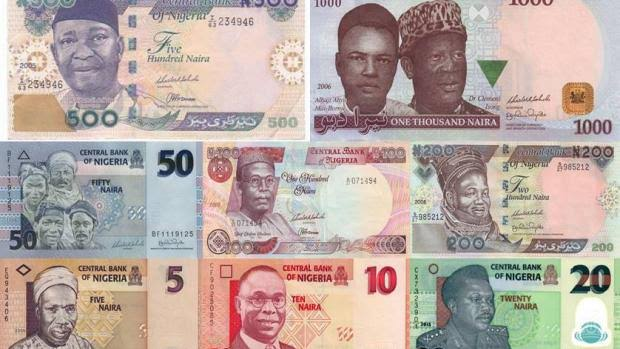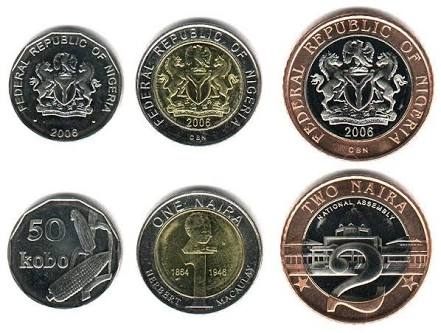# Money – Recognition of Coins and Notes (Primary 1)

MATHEMATICS

THIRD TERM

WEEK 2

PRIMARY 1

THEME – PRIMARY MEASURES

PREVIOUS LESSON – Revision on Identification, Reading and Writing Numbers up to 100 Primary 1 Primary 2 Primary 3

TOPIC – MONEY

LEARNING AREA

1. Identification of Nigerian Currencies – Naira and Coins

2. Relationships between Coins

3. Relationships between Naira

4. HomeWork

PERFORMANCE OBJECTIVES

By the end of the lesson, the pupils should have attained the following objectives (cognitive, affective and psychomotor) and should be able to –

1. recognize different denomination of Nigerian currencies.

2. recognize different denominations of Nigerian coins.

3. arrange coins in order of values.

4. express one coin in term of another.

5. differentiate between coins and notes.

ENTRY BEHAVIOUR

Nigerian currencies – Notes

INSTRUCTIONAL MATERIALS

The teacher will teach the lesson with the aid of:

1. Real coins

2. model coins or paper coins

3. Tracing paper

4. Brown paper

5. White paper

6. Crayon.

METHOD OF TEACHING – Choose a suitable and appropriate methods for the lessons.

Note – Irrespective of choosing methods of teaching, always introduce an activities that will arouse pupil’s interest or lead them to the lessons.

REFERENCE MATERIALS

1. Scheme of Work

2. 9 – Years Basic Education Curriculum

3. Course Book

4. All Relevant Material

5. Online Information

CONTENT OF THE LESSON

LESSON ONE – INTRODUCTORY ACTIVITIES

NIGERIAN NOTES AND COINS

Pupils Activities – Identification of Nigerian MoneyPupil’s Response – This is money – Nigerian money. It is called NAIRA. ₦5, ₦10, ₦20, ₦50, ₦100, ₦200, ₦100 and ₦1000.

Teacher’s Activities – What are the uses of money?

Pupil’s Response

1. It is used for buying and selling.

2. It is used for paying school fees.

3. It is used paying house rent.

4. It is used for paying transport fares, etc.

Teacher’s remark – Listen, supports and notes their points on the board or chart.

Pupil’s Activities – Identification of Nigerian CoinsTeacher’s/Pupil’s Activities – This is also Nigerian money – 50 kobo, ₦1 and ₦2.

Note – Some of the pupils are familiar with the coins while some are not.

Teacher’s remark – 50 kobo, ₦1 and ₦2 are the recent Nigerian coins.

LESSON EVALUATION

1 arrange coins in order of values.

2. different denomination of Nigerian coins and naira.

LESSON TWO – RELATIONSHIP BETWEEN COINS

Teacher’s/Pupil’s Activities

QUANTITATIVE REASONING

Sample – 50 kobo + 50 k = ₦1

EXERCISE

1. ₦1 + ₦1 = ____

2. 50 kobo + 50 kobo + ₦1 = ____

3. 50 kobo + ₦1 + 50 kobo = ____

4. 50 kobo + 50 kobo + 50 kobo + 50 kobo =

5. ₦2 = ₦1 + ____ + ____

6. ₦2 = 50 kobo + ____ + 50 kobo + ____

LESSON THREE – RELATIONSHIP BETWEEN NAIRA NOTES

Teacher’s/Pupil’s Activities

QUANTITATIVE REASONING

Sample

1. ₦5 + ₦5 = ₦10

2. ₦10 + ₦10 = ₦20

3. ₦50 + ₦50 = ₦100

4. ₦100 + ₦100 = ₦200

5. ₦200 + ₦200 + ₦200 + ₦200 + ₦200 = ____

6. ₦500 + ₦500 = ₦1000

EXERCISE

1. ₦50 = ₦20 + ₦10 + ____

2. ₦200 = ₦100 + ₦50 + ____

3. ₦500 = ₦200 + ____ + ₦200

5. 1000 = ₦500 + ____

6. ₦1000 = ₦500 + ____ + ₦100 + ____ + ____

HOMEWORK

Arrange from the smallest amount to the largest amount.

1. ₦1, ₦2 and ₦50 kobo.

2. ₦500, ₦100, ₦50, ₦100, ₦5, ₦1000, ₦200 and ₦10

PRESENTATION

To deliver the lesson, the teacher adopts the following steps:

1. To introduce the lesson, the teacher revises the previous lesson. Based on this, he/she asks the pupils some questions;

2. Leads pupils through various activities to recognize the different denominations of Nigeria coins.

Pupil’s Activities – Engage in sorting of coins into various denominations from the collection of coins.

3. Devises suitable games for pupils to play in order to recognize the coins.

Pupil’s Activities – Identify the different denominations.

CONCLUSION

• To conclude the lesson for the week, the teacher revises the entire lesson and links it to the following week’s lesson.

LESSON EVALUATION

Pupils to:

1. give a collection of coins.

2. identify some as specified by the teacher.